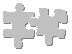#Derive Phi Exactly

Once you know that

```phi + 1 = phi * phi

```
you can apply simple algebra and find the actual value of phi.

First, combine and rearrange the terms to form a standard quadratic equation:

```   2
phi  - phi - 1 = 0

```

Now we can use the Quadratic Formula to solve for phi.

The Quadratic Formula says that when you have a standard quadratic equation, like this:

```  2
ax  + bx + c = 0

```
then you can find the value of x from the values of a, b, and c, like so:
```              _________
/ 2
-b +- \/ b  - 4ac
x = --------------------
2a

```

Going back to our equation for phi

```   2
phi  - phi - 1 = 0

```
we get these values for a, b, and c:
```a =  1
b = -1
c = -1

```

Plugging into the Quadratic Formula gives:

```                    ________________
/    2
-(-1)  +- \/ (-1)  - 4(1)(-1)
phi = -------------------------------
2(1)

_______
1 +- \/ 1 + 4
= ----------------
2

___
1 +- \/ 5
= ------------
2

```

Your pocket calculator, computer, or slide rule can tell you, with varying degrees of accuracy, that the precise value of the square root of 5 is (approximately)

```2.2360679774997...

```

```
1 + 2.2360679774997...
------------------------  ==>  1.61803398874989...
2

and
(remember a square root has two values, one positive and one negative)

1 - 2.2360679774997...
------------------------  ==>  -0.61803398874989...
2

```

This is puzzling.
The first number makes sense, because we know that the number we're looking for is bigger than one and greater than zero, that being in the nature of growth (things get larger when you multiply by phi, don't they?). But when you've finished
unfolding the Golden Rectangle, and you go backwards towards the origin, things are getting smaller and moving in the opposite direction. That's when you meet this unexpected alter ego of phi.

Hmmm... We got here because, if you add one to phi you get phi squared. What happens if we subtract one from phi? Well, multply by -1 and you've got this same inverted side of phi, once again moving backwards and down towards the Cosmic Navel.

I'll leave this question to you: We know that phi somehow embodies both addition and multiplication. We just looked at subtraction - now, what do you get if you divide one by phi?

Could it be that those seemingly primal operations of addition, subtraction, multiplication, and division are really just four faces of phi?

By the way, if

```             2
1 + phi = phi

```
then what does this equal?
```         2
phi + phi  = ?

```
```   4      5
phi  + phi  = ?

```
Or, for that matter?
```   499      500
phi    + phi    = ?

3    6        501
Did you guess phi, phi, and phi   ?

```

What we've stumbled onto here is the mathematically perfect parallel of the Fibonacci series we started out with. If you recall, a Fibonacci series is defined by each successive term being the sum of the previous two. So if you start out with one and phi, you get:

```1, phi, (phi + 1), (phi + (phi + 1)), ((phi + 1) + (phi + (phi + 1))), ...

```
Or, as we just saw:
```                 2                  3                                4
1, phi,       phi,               phi,                             phi,

```

Each term in these two series is equivalent. For instance:

```  1  = 1

phi  = phi
2
phi  = (1 + phi)
3
phi  = (phi + (1 + phi))
4
phi  = ((1 + phi) + (phi + (1 + phi)))

```
and so on. Notice that each power of phi can be calculated by addition - no multiplication required! This time there's no approximation; each successive term in the series is exactly the sum of the two previous two terms and at the same time is exactly the previous term multiplied by phi.

## The Golden Mean

[ The Golden Mean | The Graphical Derivation of Phi | Derive Phi Exactly | Unfold the Golden Rectangle ]